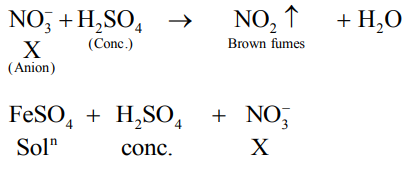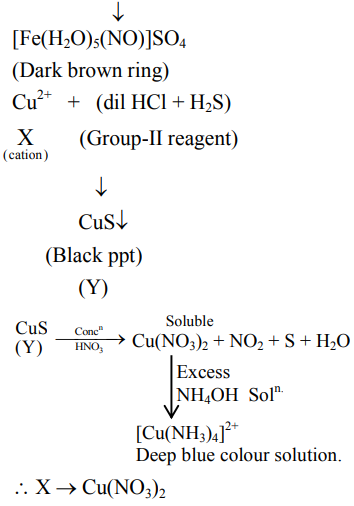# An inorganic Compound 'X' on treatment with concentrated`
Question:

An inorganic Compound ' $\mathrm{X}$ ' on treatment with concentrated $\mathrm{H}_{2} \mathrm{SO}_{4}$ produces brown fumes and gives dark brown ring with $\mathrm{FeSO}_{4}$ in presence of concentrated $\mathrm{H}_{2} \mathrm{SO}_{4}$. Also Compound ' $\mathrm{X}$ ' gives precipitate ' $\mathrm{Y}^{\prime}$, when its solution in dilute $\mathrm{HCl}$ is treated with $\mathrm{H}_{2} \mathrm{~S}$ gas. The precipitate ' $\mathrm{Y}$ ' on treatment with concentrated $\mathrm{HNO}_{3}$ followed by excess of $\mathrm{NH}_{4} \mathrm{OH}$ further gives deep blue coloured solution, Compound ' $\mathrm{X}$ ' is:

1. $\mathrm{Co}\left(\mathrm{NO}_{3}\right)_{2}$

2. $\mathrm{Pb}\left(\mathrm{NO}_{2}\right)_{2}$

3. $\mathrm{Cu}\left(\mathrm{NO}_{3}\right)_{2}$

4. $\mathrm{Pb}\left(\mathrm{NO}_{3}\right)_{2}$

Correct Option: , 3

Solution: